# Problem with electrostatics

• NicolaiTheDane

## Homework Statement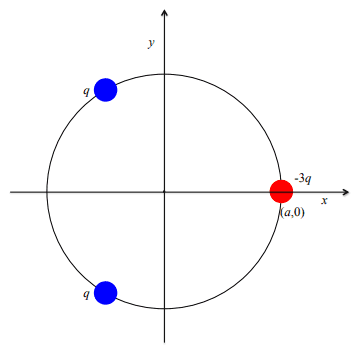I'm suppose to set a general function of the electric field, at any point in the xy plane. As the picture shows, there are 2 point charges with 1 charge, and 1 point charge with -3 charge. Each of them are equally distanced from the origin, and all 3 are evenly spaced apart.

## Homework Equations

I'm used Coulumbs law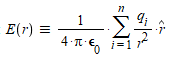## The Attempt at a Solution

First I defined all the point charge locations, along with an arbitrary point, to represent the unknown point of the function.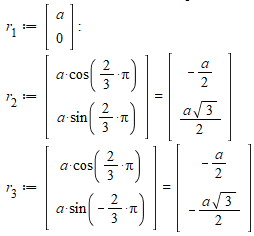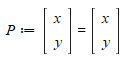Then I merely use Coumlumbs law, to add up all the expressions: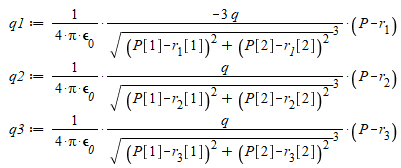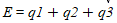The final expression isn't pretty at all though: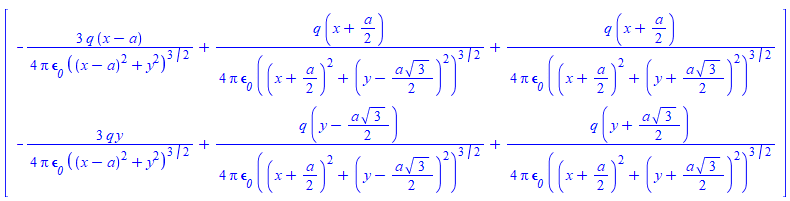The field as displayed by maple (if one fixes the fieldstrength, otherwise some arrows become very very small) looks like this: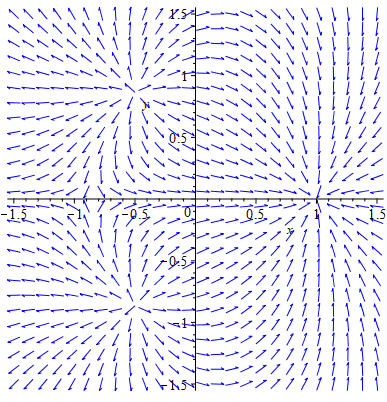Which to me looks about right. However the vector function isn't divergence free, which suggests I have messed up something. What am I doing wrong?

#### Attachments

The two dimensional function will not be divergence free, but if you included the z-component of the electric field, it would then be divergence free except at the locations of the electrical charges. ## \\ ## I didn't carefully check your equations, but they seem to be correct.

•NicolaiTheDane
The two dimensional function will not be divergence free, but if you included the z-component of the electric field, it would then be divergence free except at the locations of the electrical charges. ## \\ ## I didn't carefully check your equations, but they seem to be correct.

Ahh alright then. Thanks a bunch! :)

Ahh alright then. Thanks a bunch! :)
What is also the case is that when you draw lines of flux for a 3-D case in two dimensions, the result is inherent inaccuracies where the density of lines doesn't fall off as inverse square, etc. The line density will be ## \frac{1}{r} ## in a 2-D diagram of a 3 dimensional case if they are drawn as continuous lines.

Last edited:
And an addition to post 2: You can see this with a single point charge: If you let ## E=\frac{Q}{4 \pi \epsilon_o}(\frac{x}{(x^2+y^2)^{3/2}} \hat{i} +\frac{y}{(x^2+y^2)^{3/2}} \hat{j}) ##, the divergence is non-zero everywhere, but if you write it as ## E=\frac{Q}{4 \pi \epsilon_o}(\frac{x}{(x^2+y^2+z^2)^{3/2}} \hat{i}+\frac{y}{(x^2+y^2+z^2)^{3/2}} \hat{j}+\frac{z}{(x^2+y^2+z^2)^{3/2}} \hat{k} ) ## and compute ## \nabla \cdot E ##, you will find it is indeed zero everywhere except at ## (0,0,0) ##.